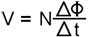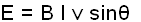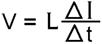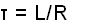Home / Electrical MCQs / Inductors MCQs with Answers

Want create site? Find Free WordPress Themes and plugins.
1. In electric circuits, ‘inductance’ is the property of a circuit which:

 A. produces a voltage in a conductor
 B. causes the circuit current to increase
 C. remains at a constant value at switch on and switch off
 D. prevents any opposition to voltage flow

2. Inductors have the effect of storing current as an electromagnetic field whenever the current increases and:

 A. storing voltage when the current decreases
 B. giving it back when the current decreases
 C. remaining constant when the current decreases
 D. storing current as resistance when the current decreases

3.
The following diagram shows the symbol for a:

 A. air-cored inductor
 B. ferrite cored indictor
 C. iron cored inductor
 D. epoxy-resin cored inductor

4. An EMF can be induced in a conductor if there is a relative movement between a conductor and:

 A. a resistance
 B. an inductor
 C. the supply resistor
 D. a magnetic field

5. Faraday’s Law of electromagnetic induction states that the value of the EMF induced in a circuit depends on the number of conductors in the circuit and the rate of change of the:

 A. magnetic flux linking the conductors
 B. electrostatic flux linking the conductors
 D. magnetic current flowing through the air-gap

6. The following formula can be used to determine the voltage induced in a conductor by a moving magnetic field:In the formula, the ø stands for the:

 A. change in magnetic voltage
 B. magnetic flux in webers
 C. time period for the magnetic change
 D. number of conductors cut by the magnetic field

7. A coil of 400 turns is cut by a magnetic field of 0.15 Wb in 4 seconds. The voltage induced in the coil will be:

 A. 25 V
 B. 60 V
 C. 15 V
 D. 40 V

8. The EMF generated in a conductor can be determined using the following formula:In the formula the θ symbol stands for the:

 A. flux density of the magnetic field in teslas
 B. velocity of the conductor in metres per second
 C. length of the conductors being cut by the magnetic field
 D. angle at which the magnetic field cuts the conductors

9. A 15 cm conductor is rotated within a magnetic field of a constant density of 0.8 Tesla. If the velocity relative to the field is 12 m per second, then the voltage generated at a cutting angle of 45º will be:

 A. 1.22 V
 B. 1.54 V
 C. 0.80 V
 D. 4.53 V

10. The inductive effect of Lenz’s law is such that the induced current will appear in such a direction that it:

 A. is always in the same direction as the change that produced it
 B. opposes the change that produced it
 C. acts to assist the change that produced it
 D. not induce a voltage in the conductor

11. Magnetic inductance occurs when a magnet:

 A. is used as a compass to find direction
 B. has been demagnetized by a physical force
 C. induces a magnetic field in another material
 D. loses its magnetism due to rough handling

12. In an electric circuit, inductance occurs when a current in one circuit causes:

 A. a short circuit in an another circuit
 B. the resistance of the circuit to collapse
 C. the supply voltage to alternate with another circuit
 D. another current to be created in that same circuit

13. The value of inductance in an inductor is a function of the size and number of turns in the coil, the magnetic effects of the core and the:

 A. flux density at which it is operating
 B. size of the induction coil
 C. type of core that the coil is using
 D. initial resistance of the conductor in the coil

14.
The photo shows:

 A. an air cored inductor
 B. a laminated iron-cored inductor
 C. a powered ferrite cored inductor
 D. a wireless inductor

15. The henry is the inductance of a closed circuit in which an EMF of 1 volt is produced when the electric current flowing in the circuit varies uniformly at the rate of:

 A. one volt per second
 B. one volt per minute
 C. one ampere per second
 D. one ampere per minute

16. The EMF generated in an inductor can be determined using the following formula:In the formula the L symbol stands for the:

 A. length of the coil in meters
 B. length of the conductors in meters
 C. amount of time since the last induction
 D. inductance of the coil in Henry

17. If the current through an inductor of 1.2 H is reduced uniformly from 6 A to 1 A in 0.4s, then the value of the induced EMF will be:

 A. 9.60 V
 B. 7.20 V
 C. 0.96 V
 D. 0.72 V

18. The current in a 150 turn coil changes from 4 amps to zero amps causing a flux change of 200 mWb. The inductance of the coil is:

 A. 1.5 H
 B. 7.5 H
 C. 4.0 H
 D. 15 H

19. The following formula can be used to determine the inductance of a coil from physical quantities.In the formula the term μo stands for the:

 A. zero reference magnetic flux
 B. relative permeability
 C. absolute permeability
 D. cross section area of the coil

20. An air cored coil with 50 turns of wire has been wound over a length of 100 mm on a plastic pipe with an outside diameter of 25 mm. The inductance of coil will be:

 A. 27.64 uH
 B. 48.68 uH
 C. 54.52 uH
 D. 61.68 uH

21. The term ‘self-inductance’ is used when a conductor has a voltage induced in it by:

 A. its own magnetic field
 B. a nearby magnetic field
 C. its own electromotive force
 D. a nearby electromotive force

22. When voltage is applied, to an inductor, a magnetic field builds up and in doing so, produces a generated voltage that:

 A. assists the voltage producing it
 B. opposes the applied voltage
 C. prevents any current flow
 D. acts to keep the circuit resistive

23. The inclusion of an iron core within a coil:

 A. prevents any build up of a magnetic field when current flow
 B. decreases the field strength produced for a given current flow
 C. increases the field strength produced for a given current flow
 D. increases the voltage flow produced for a given current flow

24. With an inductor, the value of any induced voltage depends on the:

 A. resistance of the circuit
 B. frequency of the applied voltage
 C. amount of time the current is steady
 D. rate of change of flux linkages

25. The following diagram illustrates the relative directions and values of induced voltage during ‘circuit make’ and ‘circuit break:The very high self-induced voltage occurring at ‘switch off’ is due to the:

 A. collapsing magnetic field
 B. increasing supply voltage
 C. current commencing to build up
 D. voltage and current locking into synchronism

26. When opening highly inductive circuits, it is necessary to use a bypass circuit through which the high self induced voltage may be discharged. This bypass circuit is called a:

 A. surge circuit
 B. snubber circuit
 C. magnetic release circuit
 D. power control circuit

27. Any two coils sharing a part of a magnetic field will experience:

 A. an increase of supply voltage
 B. current build-up
 C. mutual inductance to some extent
 D. some change in the number of turns

28. When inductors are placed in series, the total value of the inductance is determined by:

 A. using Ohm’s law
 B. the inverse of the sum of the inverse inductance values
 C. combining the values in parallel
 D. addition of the individual inductor values

29. The voltage across the inductor rises almost instantaneously to a maximum when switched on:

 A. but the current flow takes some time to reach its maximum value
 B. and will then decrease to zero instantly
 C. and the current flow rises at the same rate as the applied voltage
 D. and reduces to zero instantly the supply is switched off

30. An inductor is therefore said to have a time constant τ = tau) which is found from the formula:In the formula the symbol ‘L’ stands for the:

 A. length of the coil in meters
 B. inductance of the coil in Henry
 C. length of time the current flows
 D. resistance of the circuit in ohms

31. The following diagram shows a time constant curve applied to the rise of current in an inductive circuit:Three time-constants after switch on, the current will be:

 A. 63.2% of its final value
 B. 86.4% of its final value
 C. 98.2% of its final value
 D. 99.3% of its final value

32. A 0.75 H choke has an internal resistance of 20 Ω. The time constant of the choke is:

 A. 15.2 ms
 B. 26.6 ms
 C. 27.5 ms
 D. 37.5 ms

33. The energy that can be stored in the electromagnetic field around an inductor can be calculated from the following formula:In the formula the term ‘I’ stands for:

 A. current flowing in the inductor
 B. intensity of the magnetic field
 C. interval between the time constants
 D. coil inductance in henrys

34. A 6 H electromagnet with an internal resistance of 40 Ω has a current flowing through it of 5A. The energy stored in its fully charged magnetic field is:

 A. 240 J
 B. 75 J
 C. 150 J
 D. 35 J

35. Care must be taken when working with large inductors as the energy in an inductor can be discharged very fast. This will result in the:

 A. build up of coil turns
 B. electromotive effect
 C. generation of a very high voltage
 D. lines of magnetic force becoming stationary

36. Coils often have a magnetic core, such as laminated iron or ferrite added to:

 A. prevent current flow
 B. act as an insulator
 C. assist in mounting the coil
 D. increase the inductance

37. Toroidal cores of ceramic materials are popular in many areas of electronics and electrical engineering mainly because:

 A. very high value inductors are available in small physical sizes
 B. very low value inductors are available in large physical sizes
 C. they are not suitable for both a.c and d.c. applications
 D. they can be manufactured without using any windings

38.
The diagram illustrates the principle of operation of a Kettering ignition system. The operation of this system is based on, which is based on:

 A. the ceramic effect
 B. induction principles
 C. Piezo-electric principles
 D. the Hall effect

39. If the insulation on an inductor breaks down, it can present two common problems-shorted turns and:

 A. open circuits
 B. poor resistance
 C. earth faults

40. Broken conductors can occur in inductors, usually due to:

 A. insulation breakdown
 B. fusion-welded joints
 C. the current being too small
 D. coil vibration

41. The insulation resistance of inductors with more than one coil should be tested between coils and an insulation value greater than:

 A. 1 MΩ should be expected
 B. 2 MΩ should be expected
 C. 5 MΩ should be expected
 D. 10 MΩ should be expected

42. The resistance of inductors can be measured to ensure that the coil has continuity. This test ensures that the:

 A. current will continue through to the Earthing medium
 B. resistance of the coil is the correct value
 C. insulation resistance of the coil is low enough
 D. applied voltage will not be connected to the frame

43. Before putting into service, relays using induction coils, should be given an insulation resistance test, a continuity test and:

 A. a drop test
 B. an over voltage test
 C. an operational test
 D. a high current test

Did you find apk for android? You can find new Free Android Games and apps.